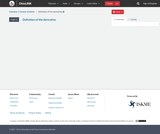# 234 Results

View
Selected filters:
• Calculus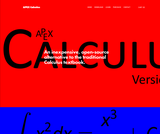Conditional Remix & Share Permitted
CC BY-NC
Rating

APEX Calculus is a calculus textbook written for traditional college/university calculus courses. It has the look and feel of the calculus book you likely use right now (Stewart, Thomas & Finney, etc.). The explanations of new concepts is clear, written for someone who does not yet know calculus. Each section ends with an exercise set with ample problems to practice & test skills (odd answers are in the back).

Subject:
Calculus
Material Type:
Textbook
Provider:
APEX: Affordable Print and Electronic TeXtbooks
Author:
Brian Heinold
Dimplekumar Chalishajar
Gregory Hartman
Troy Siemers
01/01/2014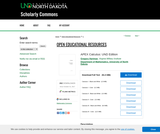Conditional Remix & Share Permitted
CC BY-NC
Rating

This text comprises a three–volume series on Calculus. The first part covers material taught in many “Calculus 1” courses: limits, derivatives, and the basics of integration, found in Chapters 1 through 6. The second text covers material often taught in “Calculus 2”: integration and its applications, along with an introduction to sequences, series and Taylor Polynomials, found in Chapters 7 through 10. The third text covers topics common in “Calculus 3” or “Multivariable Calculus”: parametric equations, polar coordinates, vector–valued functions, and functions of more than one variable, found in Chapters 11 through 15. All three are available separately for free.

Subject:
Calculus
Material Type:
Textbook
Provider:
University of North Dakota
Author:
Gregory Hartman
University of North Dakota Mathematics Department
07/01/2017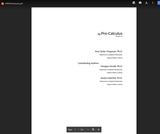Conditional Remix & Share Permitted
CC BY-NC
Rating

This text was written as a prequel to the APEXCalculus series, a three–volume series on Calculus. This text is not intended to fully prepare students with all of the mathematical knowledge they need to tackle Calculus, rather it is designed to review mathematical concepts that are often stumbling blocks in the Calculus sequence. It starts basic and builds to more complex topics. This text is written so that each section and topic largely stands on its own, making it a good resource for students in Calculus who are struggling with the supporting mathemathics found in Calculus courses. The topics were chosen based on experience; several instructors in the Applied Mathemathics Department at the Virginia Military Institute (VMI) compiled a list of topics that Calculus students commonly struggle with, giving the focus of this text. This allows for a more focused approach; at first glance one of the obvious differences from a standard Pre-Calculus text is its size.

Subject:
Calculus
Material Type:
Textbook
Author:
Amy Givler Chapman
Jessica Libertini
Meagan Herald
12/05/2019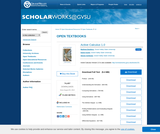Conditional Remix & Share Permitted
CC BY-NC-SA
Rating

Active Calculus is different from most existing calculus texts in at least the following ways: the text is free for download by students and instructors in .pdf format; in the electronic format, graphics are in full color and there are live html links to java applets; the text is open source, and interested instructors can gain access to the original source files upon request; the style of the text requires students to be active learners — there are very few worked examples in the text, with there instead being 3-4 activities per section that engage students in connecting ideas, solving problems, and developing understanding of key calculus concepts; each section begins with motivating questions, a brief introduction, and a preview activity, all of which are designed to be read and completed prior to class; the exercises are few in number and challenging in nature.

Subject:
Mathematics
Calculus
Material Type:
Textbook
Provider:
Grand Valley State University
Author:
David Austin
Matt Boelkins
Steve Schlicker
12/05/2019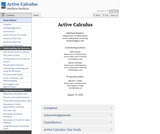Conditional Remix & Share Permitted
CC BY-NC-SA
Rating

Active Calculus is different from most existing calculus texts in at least the following ways: the text is freely readable online in HTML format and is also available for in PDF; in the electronic format, graphics are in full color and there are live links to java applets; version 2.0 now contains WeBWorK exercises in each chapter, which are fully interactive in the HTML format and included in print in the PDF; the text is open source, and interested users can gain access to the original source files on GitHub; the style of the text requires students to be active learners — there are very few worked examples in the text, with there instead being 3-4 activities per section that engage students in connecting ideas, solving problems, and developing understanding of key calculus concepts; each section begins with motivating questions, a brief introduction, and a preview activity, all of which are designed to be read and completed prior to class; following the WeBWorK exercises in each section, there are several challenging problems that require students to connect key ideas and write to communicate their understanding.

Subject:
Calculus
Material Type:
Textbook
Provider:
Grand Valley State University
Author:
David Austin
Matt Boelkins
Steve Schlicker
11/18/2021Conditional Remix & Share Permitted
CC BY-NC-SA
Rating

Active Calculus is different from most existing calculus texts in at least the following ways: the text is freely readable online in HTML format and is also available for in PDF; in the electronic format, graphics are in full color and there are live links to java applets; version 2.0 now contains WeBWorK exercises in each chapter, which are fully interactive in the HTML format and included in print in the PDF; the text is open source, and interested users can gain access to the original source files on GitHub; the style of the text requires students to be active learners — there are very few worked examples in the text, with there instead being 3-4 activities per section that engage students in connecting ideas, solving problems, and developing understanding of key calculus concepts; each section begins with motivating questions, a brief introduction, and a preview activity, all of which are designed to be read and completed prior to class; following the WeBWorK exercises in each section, there are several challenging problems that require students to connect key ideas and write to communicate their understanding.

Subject:
Calculus
Material Type:
Textbook
Author:
Austin David
Boelkins Matthew
David Austin
Matthew Boelkins
Schlicker Steven
Steven Schlicker
08/28/2020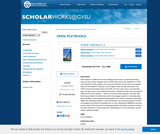Conditional Remix & Share Permitted
CC BY-NC-SA
Rating

ctive Calculus is different from most existing calculus texts in at least the following ways: the text is freely readable online in HTML format and is also available for in PDF; in the electronic format, graphics are in full color and there are live links to java applets; there are live WeBWorK exercises in each chapter, which are fully interactive in the HTML format and included in print in the PDF; the text is open source, and interested users can gain access to the original source files on GitHub; the style of the text requires students to be active learners — there are very few worked examples in the text, with there instead being 3-4 activities per section that engage students in connecting ideas, solving problems, and developing understanding of key calculus concepts; each section begins with motivating questions, a brief introduction, and a preview activity, all of which are designed to be read and completed prior to class; following the WeBWorK exercises in each section, there are several challenging problems that require students to connect key ideas and write to communicate their understanding.

Subject:
Calculus
Material Type:
Textbook
Author:
Austin David
Boelkins Matthew
David Austin
Matthew Boelkins
Schlicker Steven
Steven Schlicker
08/28/2020Conditional Remix & Share Permitted
CC BY-NC-SA
Rating

Active Calculus Multivariable is the continuation of Active Calculus to multivariable functions. The Active Calculus texts are different from most existing calculus texts in at least the following ways: the texts are free for download by students and instructors in .pdf format; in the electronic format, graphics are in full color and there are live html links to java applets; the texts are open source, and interested instructors can gain access to the original source files upon request; the style of the texts requires students to be active learners — there are very few worked examples in the texts, with there instead being 3-4 activities per section that engage students in connecting ideas, solving problems, and developing understanding of key calculus concepts; each section begins with motivating questions, a brief introduction, and a preview activity, all of which are designed to be read and completed prior to class; the exercises are few in number and challenging in nature.

Subject:
Calculus
Material Type:
Textbook
Provider:
Grand Valley State University
Author:
David Austin
Matthew Boelkins
Steven Schlicker
07/01/2018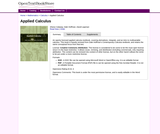Unrestricted Use
CC BY
Rating

An openly licensed applied calculus textbook, covering derivatives, integrals, and an intro to multivariable calculus. This book is heavily remixed from Dale Hoffman's Contemporary Calculus textbook, and retains the same conceptual focus from that text.

Subject:
Calculus
Material Type:
Textbook
Author:
Dale Hoffman
David Lippman
Shana Calaway
08/28/2020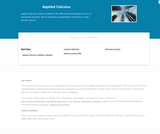Rating

Applied Calculus instructs students in the differential and integral calculus of elementary functions with an emphasis on applications to business, social and life science. Different from a traditional calculus course for engineering, science and math majors, this course does not use trigonometry, nor does it focus on mathematical proofs as an instructional method.

Subject:
Calculus
Material Type:
Full Course
Textbook
Provider:
Lumen Learning
Provider Set:
Candela Courseware
Author:
Dale Hoffman
David Lippman
Shana Calaway
06/13/2019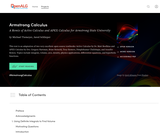Conditional Remix & Share Permitted
CC BY-NC-SA
Rating

This text is an adaptation of two very excellent open-source textbooks: Active Calculus by Dr. Matt Boelkins and APEX Calculus by Drs. Gregory Hartman, Brian Heinold, Troy Siemers, Dimplekumar Chalishajar, and Jennifer Bowen. Topics include integrals, volume, arcs, density, physics applications, differential equations, and hyperbolic functions.

1. Using Definite Integrals to Find Volume
2. Volume by The Shell Method
3. Arc Length and Surface Area
4. Density, Mass, and Center of Mass
5. Physics Applications: Work, Force, and Pressure
6. An Introduction to Differential Equations
7. Separable differential equations
8. Hyperbolic Functions

Subject:
Calculus
Material Type:
Textbook
Author:
Jared Schlieper
Michael Tiemeyer
05/10/2020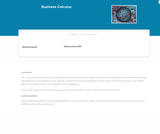Rating

This course provides an introduction to applied concepts in Calculus that are relevant to the managerial, life, and social sciences. Students should have a firm grasp of the concept of functions to succeed in this course. Topics covered include derivatives of basic functions and how they can be used to optimize quantities such as profit and revenues, as well as integrals of basic functions and how they can be used to describe the total change in a quantity over time.

Subject:
Calculus
Material Type:
Full Course
Textbook
Provider:
Lumen Learning
Provider Set:
Candela Courseware
06/13/2019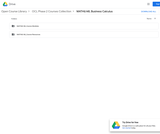Unrestricted Use
CC BY
Rating

MATH&148 is a calculus course for business students. It is designed for students who want a brief course in calculus. Topics include differential and integral calculus of elementary functions. Problems emphasize business and social science applications. Translating words into mathematics and solving word problems are emphasized over algebra. Applications are mainly business oriented (e.g. cost, revenue, and profit). Mathematical theory and complex algebraic manipulations are not mainstays of this course, which is designed to be less rigorous than the calculus sequence for scientists and engineers. Topics are presented according to the rule of four: geometrically, numerically, analytically, and verbally. That is, symbolic manipulation must be balanced with graphical interpretation, numerical examples, and writing. Trigonometry is not part of the course.

Subject:
Calculus
Material Type:
Assessment
Full Course
Syllabus
Textbook
Provider:
Washington State Board for Community & Technical Colleges
Provider Set:
Open Course Library
12/10/2019Conditional Remix & Share Permitted
CC BY-NC-SA
Rating

Published in 1991 by Wellesley-Cambridge Press, the book is a useful resource for educators and self-learners alike. It is well organized, covers single variable and multivariable calculus in depth, and is rich with applications.

In addition to the Textbook, there is also an online Instructor's Manual and a student Study Guide. Prof. Strang has also developed a related series of videos, Highlights of Calculus, on the basic ideas of calculus.

Subject:
Mathematics
Calculus
Material Type:
Full Course
Provider:
MIT
Author:
Gilbert Strang
01/01/1991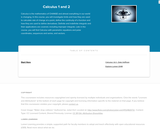Rating

Calculus is the mathematics of CHANGE and almost everything in our world is changing. In this course, you will investigate limits and how they are used to calculate rate of change at a point, define the continuity of a function and how they are used to define derivatives. Definite and indefinite integrals and their applications are covered, including improper integrals. Late in the course, you will find Calculus with parametric equations and polar coordinates, sequences and series, and vectors.

Subject:
Calculus
Material Type:
Full Course
Textbook
Provider:
Lumen Learning
Provider Set:
Candela Courseware
Author:
Dale Hoffman
David Lippman
Jeff Eldridge
Melonie Rasmussen
06/13/2019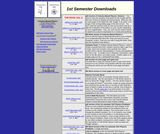Conditional Remix & Share Permitted
CC BY-SA
Rating

Calculus-Based Physics is an introductory physics textbook designed for use in the two-semester introductory physics course typically taken by science and engineering students.

Subject:
Calculus
Physics
Material Type:
Textbook
Provider:
Saint Anselm College
Author:
Jeffrey Schnick
12/05/2019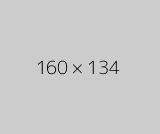Conditional Remix & Share Permitted
CC BY-NC
Rating

The Calculus I course was developed through the Ohio Department of Higher Education OER Innovation Grant. This work was completed and the course was posted in February 2019. The course is part of the Ohio Transfer Module and is also named TMM005. For more information about credit transfer between Ohio colleges and universities, please visit: www.ohiohighered.org/transfer.Team LeadJim Fowler                                         Ohio State UniversityRita Ralph                                         Columbus State Community CollegeContent ContributorsNela Lakos                                       Ohio State UniversityBart Snapp                                       Ohio State UniversityJames Talamo                                  Ohio State UniversityXiang Yan                                         Edison State Community CollegeLibrarianDaniel Dotson                                    Ohio State University                     Review TeamThomas Needham                             Ohio State UniversityCarl Stitz                                            Lakeland Community CollegeSara Rollo                                          North Central State College

Subject:
Mathematics
Calculus
Material Type:
Full Course
Provider:
Ohio Open Ed Collaborative
11/02/2020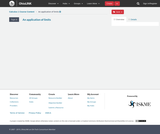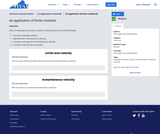Conditional Remix & Share Permitted
CC BY-NC
Rating

After completing this section, students should be able to do the following.Compute average velocity.Approximate instantaneous velocity.Compare average and instantaneous velocity.Compute instantaneous velocity.

Subject:
Calculus
Material Type:
Module
Author:
Ohio Open Ed Collaborative
11/02/2020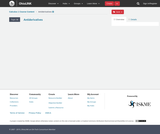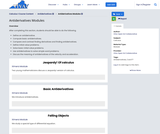Conditional Remix & Share Permitted
CC BY-NC
Rating

After completing this section, students should be able to do the following.Define an antiderivative.Compute basic antiderivatives.Compare and contrast finding derivatives and finding antiderivatives.Define initial value problems.Solve basic initial value problems.Use antiderivatives to solve simple word problems.Discuss the meaning of antiderivatives of the velocity and acceleration.

Subject:
Calculus
Material Type:
Module
Author:
Ohio Open Ed Collaborative
11/02/2020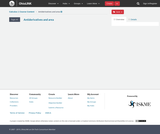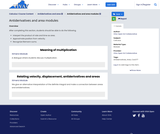Conditional Remix & Share Permitted
CC BY-NC
Rating

After completing this section, students should be able to do the following.Interpert the product of rate and time as area.Approximate position from velocity.Recognize Riemann sums.

Subject:
Calculus
Material Type:
Module
Author:
Ohio Open Ed Collaborative
11/02/2020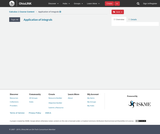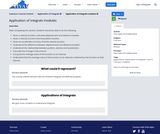Conditional Remix & Share Permitted
CC BY-NC
Rating

After completing this section, students should be able to do the following.Given a velocity function, calculate displacement and distance traveled.Given a velocity function, find the position function.Given an acceleration function, find the velocity function.Understand the difference between displacement and distance traveled.Understand the relationship between position, velocity and acceleration.Calculate the change in the amount.Compute the average value of the function on an interval.Understand that the average value of the function on an interval is attained by the function on that interval.

Subject:
Calculus
Material Type:
Module
Author:
Ohio Open Ed Collaborative
11/02/2020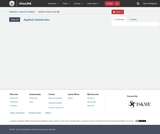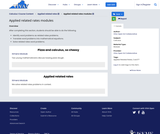Conditional Remix & Share Permitted
CC BY-NC
Rating

After completing this section, students should be able to do the following.Identify word problems as related rates problems.Translate word problems into mathematical equations.Solve related rates word problems.

Subject:
Calculus
Material Type:
Module
Author:
Ohio Open Ed Collaborative
11/02/2020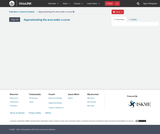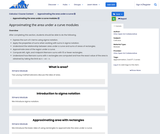Conditional Remix & Share Permitted
CC BY-NC
Rating

After completing this section, students should be able to do the following.Express the sum of n terms using sigma notation.Apply the properties of sums when working with sums in sigma notation.Understand the relationship between area under a curve and sums of areas of rectangles.Approximate area of the region under a curve.Compute left, right, and midpoint Riemann sums with 10 or fewer rectangles.Understand how Riemann sums with n rectangles are computed and how the exact value of the area is obtained by taking the limit as n→∞n→∞ .

Subject:
Calculus
Material Type:
Module
Author:
Ohio Open Ed Collaborative
11/02/2020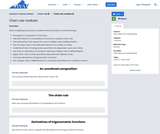Conditional Remix & Share Permitted
CC BY-NC
Rating

After completing this section, students should be able to do the following.Recognize a composition of functions.Take derivatives of compositions of functions using the chain rule.Take derivatives that require the use of multiple rules of differentiation.Use the chain rule to calculate derivatives from a table of values.Understand rate of change when quantities are dependent upon each other.Use order of operations in situations requiring multiple rules of differentiation.Apply chain rule to relate quantities expressed with different units.Compute derivatives of trigonometric functions.Use multiple rules of differentiation to calculate derivatives from a table of values.

Subject:
Calculus
Material Type:
Module
Author:
Ohio Open Ed Collaborative
11/02/2020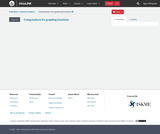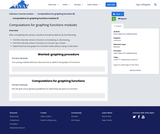Conditional Remix & Share Permitted
CC BY-NC
Rating

After completing this section, students should be able to do the following.Find the intervals where a function is increasing or decreasing.Find the intervals where a function is concave up or down.Determine how the graph of a function looks without using a calculator.

Subject:
Calculus
Material Type:
Module
Author:
Ohio Open Ed Collaborative
11/02/2020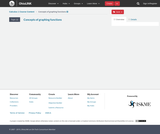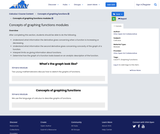Conditional Remix & Share Permitted
CC BY-NC
Rating

After completing this section, students should be able to do the following.Understand what information the derivative gives concerning when a function is increasing or decreasing.Understand what information the second derivative gives concerning concavity of the graph of a function.Interpret limits as giving information about functions.Determine how the graph of a function looks based on an analytic description of the function.

Subject:
Calculus
Material Type:
Module
Author:
Ohio Open Ed Collaborative
11/02/2020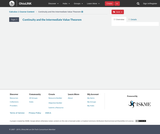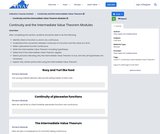Conditional Remix & Share Permitted
CC BY-NC
Rating

After completing this section, students should be able to do the following.Identify where a function is, and is not, continuous.Understand the connection between continuity of a function and the value of a limit.Make a piecewise function continuous.State the Intermediate Value Theorem including hypotheses.Determine if the Intermediate Value Theorem applies.Sketch pictures indicating why the Intermediate Value Theorem is true, and why all hypotheses are necessary.Explain why certain points exist using the Intermediate Value Theorem.

Subject:
Calculus
Material Type:
Module
Author:
Ohio Open Ed Collaborative
11/02/2020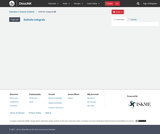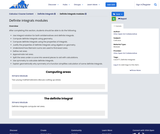Conditional Remix & Share Permitted
CC BY-NC
Rating

After completing this section, students should be able to do the following.Use integral notation for both antiderivatives and definite integrals.Compute definite integrals using geometry.Compute definite integrals using the properties of integrals.Justify the properties of definite integrals using algebra or geometry.Understand how Riemann sums are used to find exact area.Define net area.Approximate net area.Split the area under a curve into several pieces to aid with calculations.Use symmetry to calculate definite integrals.Explain geometrically why symmetry of a function simplifies calculation of some definite integrals.

Subject:
Calculus
Material Type:
Module
Author:
Ohio Open Ed Collaborative